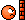# Average Velocity and Average Speed of Integral

## Homework Statement

An object moves with velocity v(t) = −t2 +1 feet per second between t = 0 and t = 2. Find the average velocity and the average speed of the object between t = 0 and t = 2

## Homework Equations

$\frac{1}{b-a} \int_a^b f'(x) dx$

avg value of a function

## The Attempt at a Solution

$\frac{1}{2-0} \int_0^2 [-t^2 + 1] dt$

$\frac{1}{2} [- \frac{t^3}{3} + t]_0^2$

$\frac{1}{2} [- \frac{8}{3} + \frac{6}{3}]$

$\frac{1}{2} [- \frac{2}{3}]$

$[- \frac{1}{3}]$

So I've got the average velocity down, but I don't see how they want me to come up with the average speed. I know that speed and velocity are similar, but speed has no direction.

The book (http://www.whitman.edu/mathematics/multivariable/" [Broken]) Instructed me to evaluate the integral without the averaging $\frac{1}{b-a}$, but I ended up with:

$- \frac{2}{3}$

But according to the solutions manual, the answer is 1

Last edited by a moderator:

The Average Speed is
$$\frac{\text{total distance traveled}}{\text{time}}$$,

see this thread for a thorough discussion:

What happens here is within the first second the object is moving with a positive velocity, but slowing down. Then, at t=1, it stops, and proceeds to move in reverse. Because you have a simple function, you should see an easy way to get the total distance here.

The Average Speed is
$$\frac{\text{total distance traveled}}{\text{time}}$$I should have had that on the top of my head! Duh!

Last edited by a moderator: